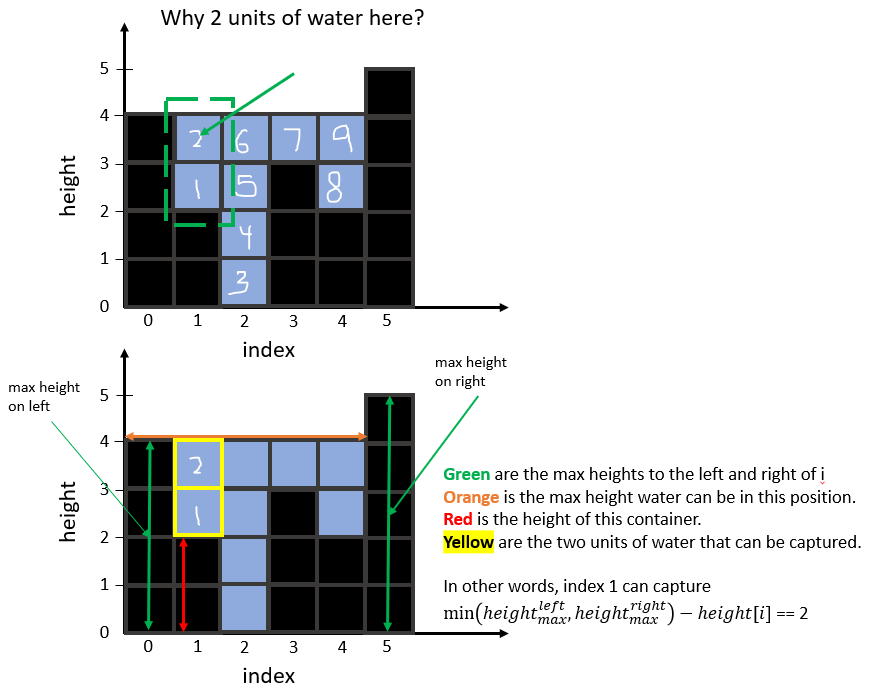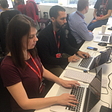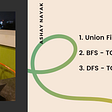# Leetcode 42 — Trapping Rain Water

This article will cover and explain 2 solutions to Leetcode 42, Trapping Rain Water. There are concepts that overlap with Leetcode 11 (Container With Most Water), and I recommend solving that before tackling this.

# Problem Overview

Given `n` non-negative integers representing an elevation map where the width of each bar is `1` , compute how much water it can trap after raining.

Constraints:

`n == height.length`

`1 <= n <= 2 * 10^4`

`0 <= height[i] <= 10^5`

A question you may have is: Does a ‘container’ require two walls and a bottom? Answer: It requires two walls (i.e. the edges don’t fill) and does not need a bottom (i.e. the x-axis is the bottom of each ‘container’).

I think it’s best to walkthrough an example to understand.

# Example

`Input: height = [4, 2, 0, 3, 2, 5]Output: 9`

To get a more in depth understanding of how those positions contains water, I’ve made diagrams that call out index `1` :This logic repeats for each position, and the total is accumulated to get the output

# Strategy, Concepts, and Solution

For us to get the total amount of water captured, we first need to understand how water is captured. Similar to Container With Most Water, the amount of water that can be contained is an area that is bounded in height by the shortest wall on the left and right. However, in this problem the heights have widths and displace water, AND we need all the containers. With that being said, I think it’s useful to take a look at the example again:

We can break down this problem by evaluating the total amount of water each position in `height`(the input elevation map) can capture. We do this by finding the maximum height to the left and right of `height[i]` . Why? Because the minimum of those heights is the highest water can be captured (if it can be). Take `index 1` from above; the highest water can be captured is 4 height units, any more and it overflows (is above the shortest wall).

Then, now that we know `height[i]'s` max height it can capture, we subtract that from `height[i]` to get the amount of water `index i`will capture. So, looking at the above figure, `height[i] = 2` and `min(height left, height right) = 4` , we have `water captured = 4 — 2 = 2` .

So, what does this look like as code? One way is to first capture the max heights to the left and right of each element:

From here, we just follow our equation to get the amount of water added per element in `height`

Notice here that I added the additional step of doing `min(0, ...)` because you could have a case where `maxLefts[i] > maxRights[i]` and `maxLefts[i] == height[i]` , so `maxRights[i] — height[i]` is negative.

Putting it all together it looks like this:

Which is O(n) time, and O(n) space. So, can we do better? What if we have a space constraint of O(1)? Here, we can use two pointers similar to Container With Most Water.

We’ll work from the outside in, with a `left` that starts at index `0` and `right` that starts at `len(height)-1`. Remember how we’re bounded by the minimum of the maximum walls to `i's` left and right? We’re going to continue to use that property but in a more efficient way.

There’s 5 things we need to track:

1. `Max Height Observed on the left`
2. `Max Height Observed on the right`
3. `A left pointer`
4. `A right pointer`
5. `The answer`

Our algorithm will have one loop, ensuring that our left and right don’t cross. We’ll increment left and right based on which is smaller. Why? It goes back to how the minimum wall height is what is the most restricting. If `height[left] < height[right]` then we know that the right side cannot be our restriction by definition. Next, we simply check if `height[left] < max height observed on the left` because if that is `False (we observed a larger height)` then we need to update our respective max height.

The two pointer solution then will read like so:

This is still O(n) time but now it is O(1) space.

Thanks for reading! I hope you enjoyed the article :))) !

--

--

--

## More from J&T Tech

Founded in 2022, J&T Tech are 2 Engineers who’s passion for teaching brought them together with one mission: To share and teach our experiences.

## Reflections on Product Engineer Role## Writing a solid Google Summer of Code Proposal## A Day in the Life: Agnes Donat, Santander## A tool to try open-source projects quickly## Success factors for building a WIN-WIN-WIN Ecosystem## #100DaysCodingChallenge## Imposter Syndrome from the Developer Perspective## Git Basic Commands## Thomas Higginson

Software Engineer working on Cognitive EW capabilities, and human that enjoys making smiles. Welcome.

## “Delete and Earn” problem using Dynamic Programming## 0/1 Knapsack## Interview Question 2— All Possible Solutions